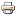# Classroom experiments and activities## Measurements using Ocular and Stage Micrometers##### Materials
• Microscope
• Ocular micrometer
• Stage micrometer
• Millimetre ruler
• Prepared slide with letter e
##### Procedure
1. Place a stage micrometer on the microscope stage, and using the lowest magnification (4X), focus on the grid of the stage micrometer.
2. Rotate the ocular micrometer by turning the appropriate eyepiece. Move the stage until you superimpose the lines of the ocular micrometer upon those of the stage micrometer. With the lines of the two micrometers coinciding at one end of the field, count the spaces of each micrometer to a point at which the lines of the micrometers coincide again (Figure 1).
3. Since each division of the stage micrometer measures 10 micrometers, and since you know how many ocular divisions are equivalent to one stage division, you can now calculate the number of micrometers in each space of the ocular scale.
4. Repeat for 10X and 40X, and 100X. Record your calculations below.

 Microscope # Value for each ocular unit at 4X Value for each ocular unit at 10X Value for each ocular unit at 40/45X Value for each ocular unit at 100X
1. Using the stage micrometer, determine the smallest length (in microns) which can be resolved with each objective. This is the measured limit of resolution for each lens. Compare this value to the theoretical limit of resolution calculated on the basis of the numerical aperture of the lens and a wavelength of 450 nm (blue light).
2. Using the calculated values for your ocular micrometer, determine the dimensions of the letter e found on your microscope slide, and add the dimensions to your drawing in Exercise 1.1. Use a millimetre ruler to measure the letter e directly and compare with the calculated values obtained through the microscope.

Notes

To measure an object seen in a microscope, an ocular micrometer serves as a scale or rule. This is simply a disc of glass upon which equally spaced divisions are etched. The rule may be divided into 50 subdivisions, or more rarely 100 subdivisions. To use the ocular micrometer, calibrate it against a fixed and known ruler, the stage micrometer. Stage micrometers also come in varying lengths, but most are 2 mm long and subdivided into 0.01 mm (10 micrometer) lengths. Each objective will need to be calibrated independently. To use, simply superimpose the ocular micrometer onto the stage micrometer and note the relationship of the length of the ocular to the stage micrometer (Refer to Figure 1.7). Note that at different magnifications, the stage micrometer changes, but the ocular micrometer is fixed in dimension. In reality, the stage micrometer is also fixed, and what is changing is the power of the magnification of the objective.

Reference:

Cell Biology Laboratory Manual

Dr. William H. Heidcamp, Biology Department, Gustavus Adolphus College,
St. Peter, MN 56082 -- cellab@gac.edu